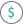#DB (PQL - xl)

This function calculates the depreciation of an asset for a specified period using the fixed‑declining balance method

• Library: PQL \ Spreadsheet \ Financial
• Compatibility: Any content (regardless of data source) in the Tabulate spreadsheet module

#### Syntax

DB(cost, salvage, life, period, OPTIONAL month)

##### Function Arguments
 Name Description Type Optional cost Initial cost of the asset Number salvage Value at the end of the depreciation period Number life Number of periods over which the asset is being depreciated Number period Period for which you want to calculate the depreciation; use the same units as the life argument Number month Number of months in the first year; if omitted, the calculation uses 12 months Number Y